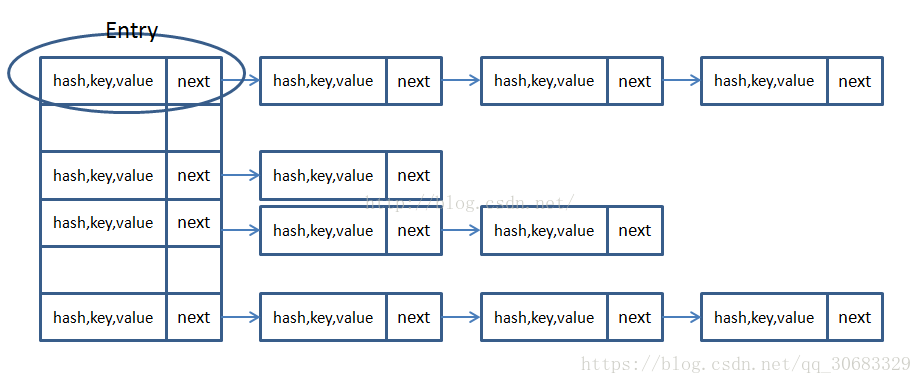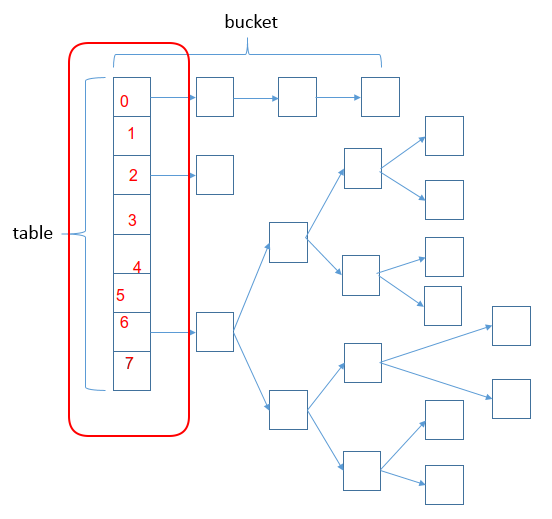以最简单的方式讲HashMap以最简单的方式讲HashMapHashMap可以说是面试中最常出现的名词，这次头条的一面，第一个问的问题就是HashMap。所以就让我们来探讨下HashMap吧。

1. HashMap的储存结构
2. HashMap中的Hash
3. HashMap是怎么保存数据的
4. HashMap的扩容操作
5. HashMap的线程安全问题

HashMap的储存结构

jdk1.7的存储结构jdk1.8的储存结构Hash

public V put(K key, V value) {
return putVal(hash(key), key, value, false, true);
}

• JDK1.8
static final int hash(Object key) {
int h;
return (key == null) ? 0 : (h = key.hashCode()) ^ (h >>> 16);
}

HashMap保存数据

public HashMap(int initialCapacity, float loadFactor) ;
• initialCapacity：初始化容量默认是16
• capacity：容量，通过initCapacity计算出一个大于或者等于initCapacity且为2的幂的值
1. 未产生hash冲突
// n是HashMap的大小，Hash为key的hash值，tab为如下图中的table，i代表储存的位置
int i;
// 为null代表此位置为空的
if ((p = tab[i = (n - 1) & hash]) == null)
tab[i] = newNode(hash, key, value, null);例如：当某一hash值与（n-1）相与的结果是3，那么就将这个这个table的第3号的位置。

2. 产生hash冲突但是如果当我们得到的hash值一样或者说相与的结果的table位置已经存在一个值了，那么我们应该怎么去储存呢？
• 当key与table[i]的所有key进行equals比较，如果相同则直接更新覆盖value。
• 假如key进行equals比较不相同，则进行元素的插入操作（在jdk1.7中是链表的插入，在jdk1.8中既有链表的插入操作也有红黑树的操作）。

HashMap保存数据的JKD1.8源代码看源代码能够更好的理解HashMap的put操作

final V putVal(int hash, K key, V value, boolean onlyIfAbsent,
boolean evict) {
Node<K,V>[] tab; Node<K,V> p; int n, i;
// 假如table是空的或者说长度为0，则进行扩容
if ((tab = table) == null || (n = tab.length) == 0)
n = (tab = resize()).length;
// 假如桶中的元素是空的，则直接将元素放在桶中【使用(n - 1) & hash]判断放的位置】
if ((p = tab[i = (n - 1) & hash]) == null)
tab[i] = newNode(hash, key, value, null);
// 假如桶中已经存在这个元素
else {
Node<K,V> e; K k;
// 假如桶中的第一个元素p的hash值，key与要存的值相等
if (p.hash == hash &&
((k = p.key) == key || (key != null && key.equals(k))))
e = p;// 使用e来记录p
// TreeNode 代表红黑树节点
// 假如key不相等，则将元素放入红黑树节点中
else if (p instanceof TreeNode)
e = ((TreeNode<K,V>)p).putTreeVal(this, tab, hash, key, value);
// 假如p为链表节点
else {
// 进行链表查找
for (int binCount = 0; ; ++binCount) {
// 假如next为空【代表达到链表末尾】
if ((e = p.next) == null) {
// 在末尾插入新的节点
p.next = newNode(hash, key, value, null);
// 如果链表长度达到阀值，则转化为红黑树
if (binCount >= TREEIFY_THRESHOLD - 1) // -1 for 1st
treeifyBin(tab, hash);
// 插入元素后跳出循环
break;
}
// 在链表中也会遇到key一样的元素，则时候就跳出循环
if (e.hash == hash &&
((k = e.key) == key || (key != null && key.equals(k))))
// 此时e为链表中key相等的元素
break;
p = e;
}
}
// e不为nul，代表要相同的元素
if (e != null) { // existing mapping for key
V oldValue = e.value;
// 如果onlyIfAbsent为false或者旧值为空，则进行更新
// 在源码中onlyIfAbsent默认是false
if (!onlyIfAbsent || oldValue == null)
e.value = value;
afterNodeAccess(e);
// 返回旧值
return oldValue;
}
}
// modeCount代表HashMap在结构上面被修改的次数
++modCount;
// 加入大小大于阀值则进行扩容
if (++size > threshold)
resize();
afterNodeInsertion(evict);
return null;
}

HashMap的扩容操作

HashMap进行扩容时，会完全新建一个桶，我们从上面了解到桶就是数组，而数组是没办法自动扩容的，所以我们需要用一个新的数组来代替前面的桶。而当HashMap进行扩容是，阀值会变成原来的两倍容量也会变成原来的两倍

1. JDK1.7 的rezise()
void resize(int newCapacity) {   //传入新的容量
//table为扩容前的Entry数组
Entry[] oldTable = table;
int oldCapacity = oldTable.length;
// 如果扩容前的数组大小如果已经达到最大(2^30)
if (oldCapacity == MAXIMUM_CAPACITY) {
//修改阈值为int的最大值(2^31-1)，这样以后就不会扩容了
threshold = Integer.MAX_VALUE;
return;
}

// 新建一个Entry数组
Entry[] newTable = new Entry[newCapacity];
//将数据转移到新的Entry数组里
transfer(newTable);
// 修改table的指向对象
table = newTable;
threshold = (int) (newCapacity * loadFactor);//修改阈值
}

void transfer(Entry[] newTable) {
Entry[] src = table;                   //src引用了旧的Entry数组
int newCapacity = newTable.length;
// 遍历旧的Entry数组
for (int j = 0; j < src.length; j++) {
Entry<K, V> e = src[j];
// 如果此位置存在元素
if (e != null) {
// for循环过后，旧的Entry数组就不再引用任何对象
src[j] = null;
// 遍历链表
do {
// 获得链表中的下一个元素
Entry<K, V> next = e.next;
// 重新计算数据保存位置
int i = indexFor(e.hash, newCapacity);
// 在jdk1.7中是头部插入，此时e.next指向新的数组位置newTable[i]
e.next = newTable[i];
// 将newTable指向e
newTable[i] = e;
// 访问下一个Entry链上的元素
e = next;
} while (e != null);
}
}
}
static int indexFor(int h, int length) {
return h & (length - 1);
}
1. JDK1.8 的rezise()

final Node<K,V>[] resize() {
Node<K,V>[] oldTab = table;
// 获得table的大小，并将其长度赋值给oldCap
int oldCap = (oldTab == null) ? 0 : oldTab.length;
// 阀值赋值
int oldThr = threshold;
int newCap, newThr = 0;
// 如果table不为空
if (oldCap > 0) {
// 数组大小大于(2^30)
if (oldCap >= MAXIMUM_CAPACITY) {
// 修改阈值为int的最大值(2^31-1)，这样以后就不会扩容了
threshold = Integer.MAX_VALUE;
return oldTab;
}
// newCap = oldCap << 1新的容量为以前的两倍
// 当新的table长度没有超过最导致，且以前的table长度大于16，则进行阀值更新
else if ((newCap = oldCap << 1) < MAXIMUM_CAPACITY &&
oldCap >= DEFAULT_INITIAL_CAPACITY)
// 阀值扩大成两倍
newThr = oldThr << 1; // double threshold
}
// 如果table为空，且阀值大于0
else if (oldThr > 0) // initial capacity was placed in threshold
// 则新的容量大小为阀值
newCap = oldThr;

// 假如table为空切阀值小于等于0，则初始化阀值，和table
else {               // zero initial threshold signifies using defaults
// 新的table长度为16
newCap = DEFAULT_INITIAL_CAPACITY;
// 新的阀值为负载因子【0.75】*16
newThr = (int)(DEFAULT_LOAD_FACTOR * DEFAULT_INITIAL_CAPACITY);
}
if (newThr == 0) {
float ft = (float)newCap * loadFactor;
newThr = (newCap < MAXIMUM_CAPACITY && ft < (float)MAXIMUM_CAPACITY ?
(int)ft : Integer.MAX_VALUE);
}
threshold = newThr;
/*
*以上都是进行初始化操作，目的是扩大容量，或则初始化HashMap
*下面便是重新存放元素操作
*/

@SuppressWarnings({"rawtypes","unchecked"})
Node<K,V>[] newTab = (Node<K,V>[])new Node[newCap];
table = newTab;
if (oldTab != null) {
for (int j = 0; j < oldCap; ++j) {
Node<K,V> e;
// 假如oldTab[j]中含有元素
if ((e = oldTab[j]) != null) {
oldTab[j] = null;
// 假如没有下一个元素，也就是oldTab[j]中只有e一个元素
if (e.next == null)
// 重新选择空间
newTab[e.hash & (newCap - 1)] = e;
// 假如有下一个元素，且该节点为红黑树节点
else if (e instanceof TreeNode)
// 将该节点进行rehash后，放到新的地方
((TreeNode<K,V>)e).split(this, newTab, j, oldCap);

/**
* 在JDK1.8中不像JDK1.7一样重新进行hash值计算，而是利用了一个规律：
* 假如e.hash & oldCap为0，那么该元素的引索位置没有变
* 假如e.hash & oldCap为1，那么该元素的引索位置为原引索+oldCap
*/
// 假如有下一个元素，但该节点为链表节点
else { // preserve order
Node<K,V> loHead = null, loTail = null;
Node<K,V> hiHead = null, hiTail = null;
Node<K,V> next;
do {
next = e.next;
if ((e.hash & oldCap) == 0) {
if (loTail == null)
else
loTail.next = e;
loTail = e;
}
else {
if (hiTail == null)
else
hiTail.next = e;
hiTail = e;
}
} while ((e = next) != null);
if (loTail != null) {
loTail.next = null;
}
if (hiTail != null) {
hiTail.next = null;
newTab[j + oldCap] = hiHead;
}
}
}
}
}
return newTab;
}

HashMap的线程安全问题

1. 多线程put()操作

1. 多线程resize()操作

HashMap其实并不是很难，我们主要是要理解它储存元素的思想与方法。而通过源代码，我们能够更好的理解设计的理念